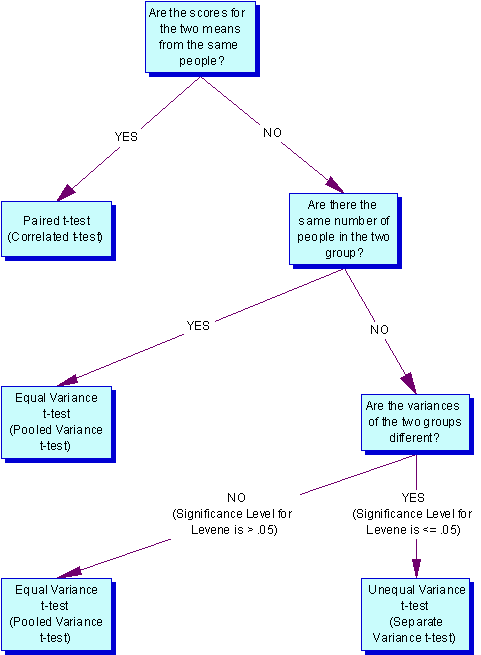# T-Test (Mean, Proportion)

The t test is one type of inferential statistics. It is used to determine whether there is a significant difference between the means of two groups. With all inferential statistics, we assume the dependent variable fits a normal distribution. When we assume a normal distribution exists, we can identify the probability of a particular outcome. We specify the level of probability (alpha level, level of significance, p) we are willing to accept before we collect data (p < .05 is a common value that is used). After we collect data we calculate a test statistic with a formula. We compare our test statistic with a critical value found on a table to see if our results fall within the acceptable level of probability.

When the difference between two population averages is being investigated, a t test is used. In other words, a t test is used when we wish to compare two means (the scores must be measured on an interval or ratio measurement scale). We would use a t test if we wished to compare the reading achievement of boys and girls. With a t test, we have one independent variable and one dependent variable. The independent variable (gender in this case) can only have two levels (male and female). The dependent variable would be reading achievement. If the independent had more than two levels, then we would use a one-way analysis of variance (ANOVA).

The test statistic that a t test produces is a t-value. Conceptually, t-values are an extension of z-scores. In a way, the t-value represents how many standard units the means of the two groups are apart.

With a t test, the researcher wants to state with some degree of confidence that the obtained difference between the means of the sample groups is too great to be a chance event and that some difference also exists in the population from which the sample was drawn. In other words, the difference that we might find between the boys’ and girls’ reading achievement in our sample might have occurred by chance, or it might exist in the population. If our t test produces a t-value that results in a probability of .01, we say that the likelihood of getting the difference we found by chance would be 1 in a 100 times. We could say that it is unlikely that our results occurred by chance and the difference we found in the sample probably exists in the populations from which it was drawn.

ASSUMPTIONS UNDERLYING THE T TEST

• The samples have been randomly drawn from their respective populations
• The scores in the population are normally distributed
• The scores in the populations have the same variance (s1=s2) Note: We use a different calculation for the standard error if they are not.

### THREE TYPES OF T TESTS1. Pair-difference t test (a.k.a. t-test for dependent groups, correlated t test) df= n (number of pairs) -1

This is concerned with the difference between the average scores of a single sample of individuals who are assessed at two different times (such as before treatment and after treatment). It can also compare average scores of samples of individuals who are paired in some way (such as siblings, mothers, daughters, persons who are matched in terms of a particular characteristics).

1. t test for Independent Samples (with two options)

This is concerned with the difference between the averages of two populations. Basically, the procedure compares the averages of two samples that were selected independently of each other, and asks whether those sample averages differ enough to believe that the populations from which they were selected also have different averages. An example would be comparing math achievement scores of an experimental group with a control group.

• Equal Variance (Pooled-variance t-test) df=n (total of both groups) -2 Note: Used when both samples have the same number of subject or when s1=s2 (Levene or F-max tests have p > .05).
• Unequal Variance (Separate-variance t test) df dependents on a formula, but a rough estimate is one less than the smallest group Note: Used when the samples have different numbers of subjects and they have different variances —  s1<>s2 (Levene or F-max tests have p < .05).

HOW DO I DECIDE WHICH TYPE OF T TEST TO USE?## 2 thoughts on “T-Test (Mean, Proportion)”

error: Content is protected !!